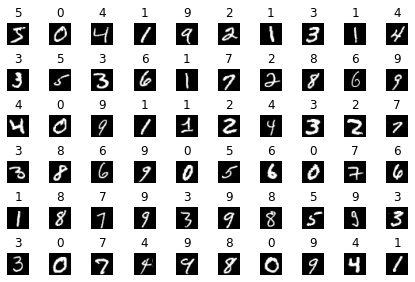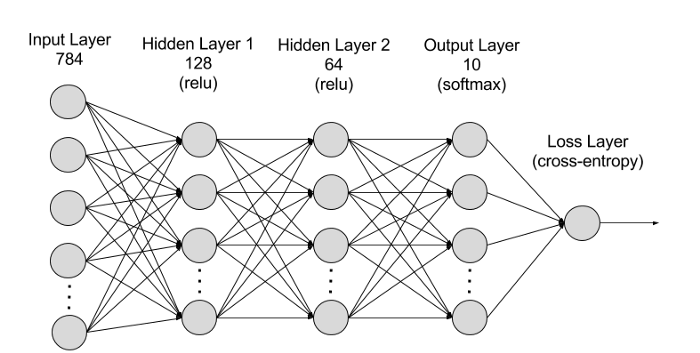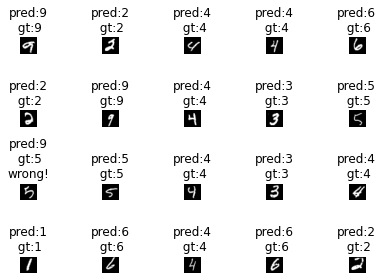In :
import torch
import torchvision
import matplotlib.pyplot as plt

import torch.nn as nn
import torch.optim as optim

Let's look at the data

In :
dataset_save_path = '../data/'

Extracting ../data/MNIST/raw/train-images-idx3-ubyte.gz to ../data/MNIST/raw

Extracting ../data/MNIST/raw/train-labels-idx1-ubyte.gz to ../data/MNIST/raw

Extracting ../data/MNIST/raw/t10k-images-idx3-ubyte.gz to ../data/MNIST/raw

Extracting ../data/MNIST/raw/t10k-labels-idx1-ubyte.gz to ../data/MNIST/raw

In :
print(dataset_train.data.shape)
print(dataset_train.data.max())

print(dataset_train.targets.shape)
print(dataset_train.targets.max())
torch.Size([60000, 28, 28])
tensor(255, dtype=torch.uint8)
torch.Size()
tensor(9)
In :
figure = plt.figure()
num_of_images = 60
for index in range(num_of_images):
plt.subplot(6, 10, index+1)
plt.axis('off')
plt.imshow(dataset_train.data[index,:,:], cmap='gray')
plt.title(int(dataset_train.targets[index]))
plt.tight_layout()Get the mean and std of the data

In :
dataset_01 = dataset_train.data.double()/255
print(dataset_01.max())
print(torch.mean(dataset_01))
print(torch.std(dataset_01))
tensor(1., dtype=torch.float64)
tensor(0.1307, dtype=torch.float64)
tensor(0.3081, dtype=torch.float64)

Prepare the data

In :
# function that acts on the data as a pre-processing stage
mnist_transform = torchvision.transforms.Compose([
torchvision.transforms.ToTensor(), # transforms the dataset to tensor from PIL/numpy + scale: [0,255] -> [0,1]
torchvision.transforms.Normalize(0.1307, 0.3081) # mean & std of train set
])

# Note: this transformation mnist_transform will be activated only upon dataloader call
transform=mnist_transform)
transform=mnist_transform)

DataLoader: Combines a dataset and a sampler, and provides an iterable over the given dataset.

In :
batch_size_train = 64
batch_size_test = 1000 # the test batch size is larger because this is less compute intensive

# shuffle: set to True to have the data reshuffled at every epoch

Build a NNIn :
input_size = 28*28
hidden_sizes = [128, 64]
output_size = 10 # Num classes

model = nn.Sequential(nn.Flatten(),
nn.Linear(input_size, hidden_sizes),
nn.ReLU(),
nn.Linear(hidden_sizes, hidden_sizes),
nn.ReLU(),
nn.Linear(hidden_sizes, output_size),
nn.Softmax(dim=1))
print(model)
Sequential(
(0): Flatten(start_dim=1, end_dim=-1)
(1): Linear(in_features=784, out_features=128, bias=True)
(2): ReLU()
(3): Linear(in_features=128, out_features=64, bias=True)
(4): ReLU()
(5): Linear(in_features=64, out_features=10, bias=True)
(6): Softmax(dim=1)
)

Get the device on which we will work

In :
print(f" is cuda available: {torch.cuda.is_available()}")
device = torch.device("cuda" if torch.cuda.is_available() else "cpu")
is cuda available: True

We need to move the weights and data to the GPU...

In :
model.to(device)
Out:
Sequential(
(0): Flatten(start_dim=1, end_dim=-1)
(1): Linear(in_features=784, out_features=128, bias=True)
(2): ReLU()
(3): Linear(in_features=128, out_features=64, bias=True)
(4): ReLU()
(5): Linear(in_features=64, out_features=10, bias=True)
(6): Softmax(dim=1)
)

• model.eval() will notify all your layers that you are in eval mode, that way, batchnorm or dropout layers will work in eval mode instead of training mode.
• torch.no_grad() impacts the autograd engine and deactivate it. It will reduce memory usage and speed up computations but you won’t be able to backprop (which you don’t want in an eval script).
In :
model.eval()
correct = 0
total = 0
# Move batch to device
images = images.to(device)
labels = labels.to(device)

outputs = model(images)
predicted = torch.argmax(outputs.data, dim=1)
total += labels.size(0)
correct += int((predicted == labels).sum())
print(f"Accuracy of the network on {dataset_test.data.shape} test images: {100 * correct / total}%")

Accuracy of the network on 10000 test images: 8.24%

Build the train loop

In :
criterion = nn.CrossEntropyLoss()

epochs = 3
for e in range(epochs):
model.train()
epoch_sum_loss = 0

# Run mini-batch
# Move batch to davice
images = images.to(device)
labels = labels.to(device)

# Training pass

output = model(images)
loss = criterion(output, labels)

#This is where the model learns by backpropagating
loss.backward()

#And optimizes its weights here
optimizer.step()

epoch_sum_loss += loss
print(f"Epoch {e} - Training epoch mean loss: {epoch_sum_loss/len(train_loader)}")
Epoch 0 - Training epoch mean loss: 1.5766130685806274
Epoch 1 - Training epoch mean loss: 1.5176198482513428
Epoch 2 - Training epoch mean loss: 1.5053908824920654

Test the final model

In :
Accuracy of the network on 10000 test images: 96.2%
In :
images, labels = dataiter.next()

outputs = model(images.to(device))

predicted = torch.argmax(outputs, dim=1)

figure = plt.figure()
num_of_images = 20
for index in range(num_of_images):
plt.subplot(4, 5, index+1)
plt.axis('off')
plt.imshow(images[index,0,:,:], cmap='gray')
prd = int(predicted[index])
gt = int(labels[index])
plt.title(f"pred:{prd}\n gt:{gt}" +"\nwrong!"*(prd!=gt))
plt.tight_layout()